# Introduction to AVL Trees (With Examples)

AVL trees are self-balancing Binary Search Trees (BST) that was invented by Adelson, Velski and Landis. Let’s consider the following:

##### 1. AVL Tree Balance Factor

In AVL trees, the difference between the depths of the left and right sub-trees should be at most 1 for every sub-tree.

Take for example the two trees in Figure 1a and 1b.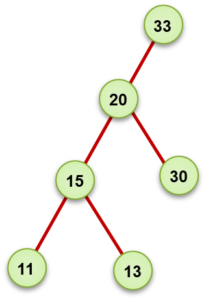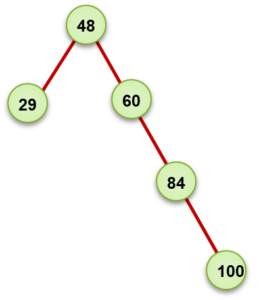Figure 1a Figure 1b Balance Factor = 3 – 0 = 3 Balance Factor = 3 – 1 = 2

In Figure 1a, consider the root node 33. The right sub-tree has a height of 3 and the left subtree has a height of  0. In this case, the difference between the height of the left subtree and the height of the right subtree is 3 – 0 = 3

In Figure 1b, consider the root node, 48. The right subtree has a height of 1 and the left subtree has a height of 3. In this case, the difference between the height of the left subtree and the height of the right subtree is 3 – 1 = 2.

In both cases, we say that the tree is not balanced.

This difference is called the Balance Factor and is given by:

Balance Factor = height(left sub-tree)  – height (right sub-tree)

Note that the balance factor cannot be negative. You always need to subtract the bigger number from the smaller number

Each time an AVL tree becomes unbalanced(balancing factor is more than 1) due to insertion or deletion, we need to perform a rotation to make the tree balanced. Rotation is also called rebalancing.

##### 2. How to Perform AVL Tree Rotations

The different kinds of rotations that can be performed in an AVL tree to make is balanced includes:

• Left Rotation
• Right Rotation
• Left-Right Rotation
• Right-Left Rotation

We would discuss these four types of rotations with examples.

Left Rotation

This is an example of simple rotation and is performed in just one step where the root node of the tree moved to the left. This is illustrated in Figure 2a, 2b and 2c.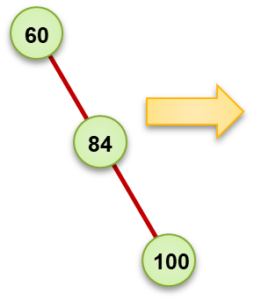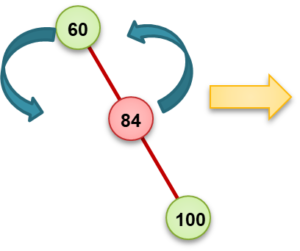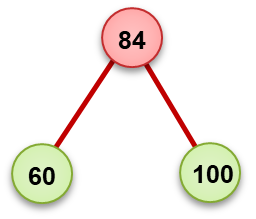Figure 2a Figure 2b Figure 2c Unbalanced Tree (BST) Left Rotation Balanced Tree (AVL Tree)

During a left rotation, the element at the root position(60) becomes the left subtree, while the element previously at the right subtree(84) moves to the root position. The right subtree of 84 remains unchanged.

Right Rotation

This is also an example of simple rotation performed in just one step. In the case of right rotation, the root node moves to the right. This is shown in Figure 3a, 3b and 3c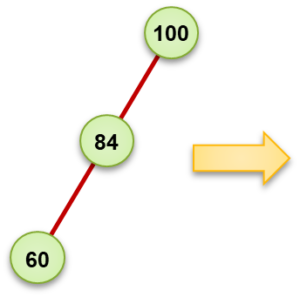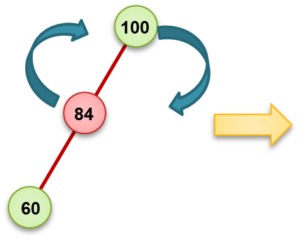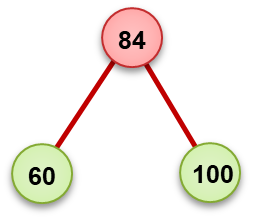Figure 3a Figure 3b Figure 3c Unbalanced Tree (BST) Left Rotation Balanced Tree (AVL Tree)

During a left rotation as shown, the element at the root position(100) becomes the right subtree, while the element previously at the left subtree(84) moves to the root position. The right subtree of 84 remains unchanged.

Left-Right Rotation

This is an example of double-rotation where a left rotation is performed followed by a right rotation.  This is required if the unbalanced is caused by the right subtree of the left subtree. This is illustrated in Figure 4a to 4e

 State Action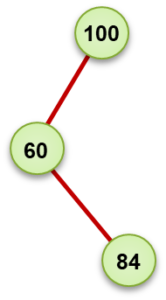Figure 4a Insertion of the node 84 as the right subtree of 60 makes the tree unbalanced. This is because of the right subtree fo the left subtree. Therefore we need to perform a left-right rotation to make the tree balanced again.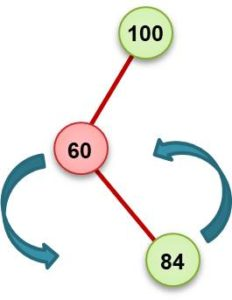Figure 4b The first step is to perform a left rotation on the node 60. This would make 84 the left subtree of 100 and 60 becomes the left subtree of 84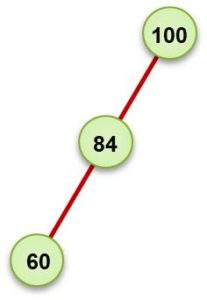Figure 4c At this point, the tree is still unbalanced at the root node 100. This is because of the left subtree of the left subtree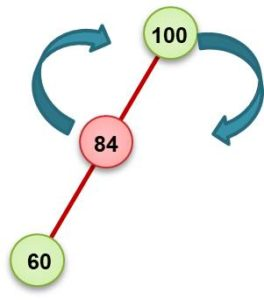Figure 4d We now perform a right rotation. This means moving 84 to the root node and moving the root node (100) as the right subtree.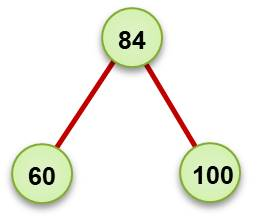Figure 4e The tree is now balanced.

Right-Left Rotation

This is also an example of double-rotation where a right rotation is performed followed by a left rotation.  This is required if the unbalanced is caused by the left subtree of the right subtree. This is illustrated in Figure 5a to 5e

 State Action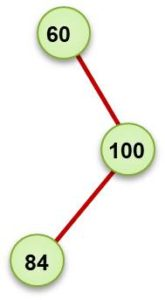Figure 5a Insertion of the node 84 as the leftsubtree of 100 makes the tree unbalanced. This is because of the left subtree fo the right subtree. Therefore we need to perform a right-left rotation to make the tree balanced again.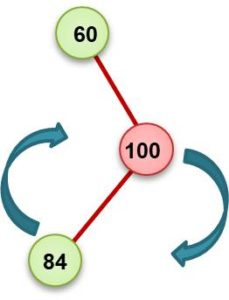Figure 5b The first step is to perform a right rotation on the node 100. This would make 84 the right subtree of 60. And 100 becomes the right subtree of 84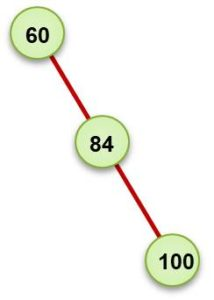Figure 5c At this point, the tree is still unbalanced at the root node 60. This is because of the right subtree of the right subtree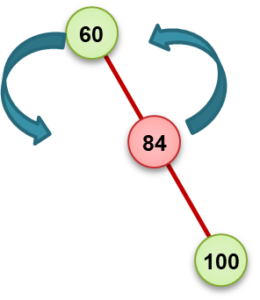Figure 5d We now perform a left rotation. This means moving 84 to the root node and moving the root node (60) as the left subtree.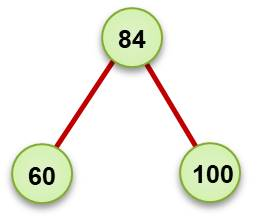Figure 5e The tree is now balanced.

This is how to perform rotations on AVL trees.

##### 3. Check these Data Structure and Algorithms Tutorials#### kindsonthegenius

Kindson Munonye is currently completing his doctoral program in Software Engineering in Budapest University of Technology and Economics

View all posts by kindsonthegenius →